# Complex Exponentials and Trig Identities

Recall that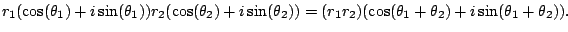The angles add. You've seen something similar before: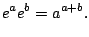This connection between exponentiation and (4.4) gives us an idea!

If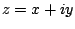is a complex number, define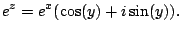We have just written polar coordinates in another form. It's a shorthand for the polar form of a complex number: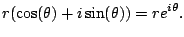Theorem 4.4.1   If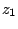,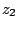are two complex numbers, then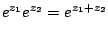Proof.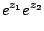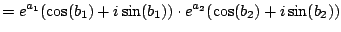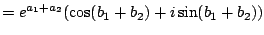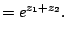Here we have just used (4.4).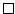The following theorem is amazing, since it involves calculus.

Theorem 4.4.2   If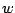is a complex number, then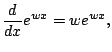for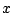real. In fact, this is even true fora complex variable (but we haven't defined differentiation for complex variables yet).

Proof. Write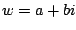.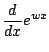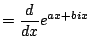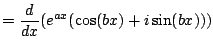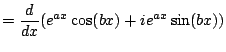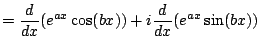Now we use the product rule to get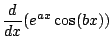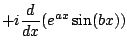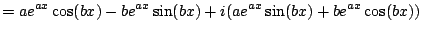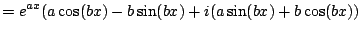On the other hand,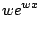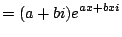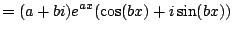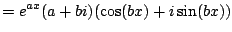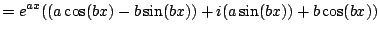Wow!! We did it!That Theorem 4.4.2 is true is pretty amazing. It's what really gets complex analysis going.

Example 4.4.3   Here's another fun fact: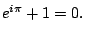Solution. By definition, have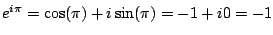.

Subsections
William Stein 2006-03-15